Courses

# Math Rules For GMAT GMAT Notes | EduRev

## Quantitative Aptitude for GMAT

Created by: Wizius Careers

## GMAT : Math Rules For GMAT GMAT Notes | EduRev

The document Math Rules For GMAT GMAT Notes | EduRev is a part of the GMAT Course Quantitative Aptitude for GMAT.
All you need of GMAT at this link: GMAT

NUMBER PROPERTIES

The most powerful EMPOWER tactic for the Quantitative section of the GMAT

WHY learn Number Properties:

1. Number properties occur on approximately 10 questions, so they have a signiﬁcant impact on your Quant Score.
2. NPs show up heavily in DS questions, so knowing NPs will lead to greater success on DS questions.
3. Knowing NPs will give you additional (often faster) ways to answer questions besides conventional math approaches. This knowledge will work well with TEST IT to help you to avoid pacing problems.

MAJOR NUMBER PROPERTIES:

(you will learn additional minor NPs in your EMPOWER Training and Quizzes and in the GMAC Ofﬁcial Guide).

even + even = even
even + odd = odd
odd + odd = even
even x even = even
even x odd = even
odd x odd = odd
positive x negative = negative
negative x negative = positive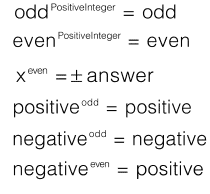Example: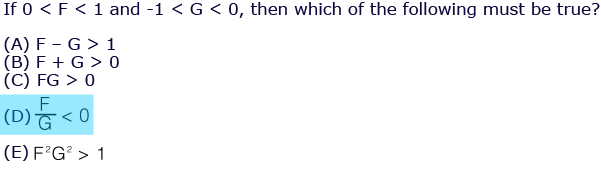MATH RULES

WHY you should know math rules:

1. They affect EVERY question in the Quant section.
2. They will provide you with necessary content knowledge and faster ways to do the necessary calculations.

RATIOS:

Ratios appear on the GMAT in 5-6 questions (about 3 problem solving and 3 DS questions) and these questions usually count! These questions require ﬂexibility on your part (sometimes you must do math, sometimes you must use TEST IT).
Ratios can come in many formats, so it’s important to understand that all of the following examples MEAN THE SAME THING.

Example:

“2 men to 5 women” = 2:5 = Men/Women = 2/5 = 0.4 = 40% = “2 out of 7 people are men”
2 important things to remember about ratios:

1. Ratios are all about MULTIPLES.
2. Ratios tend to be Sufﬁcient.

Example:

Men : Women = 2 : 5
x 1        Men = 2; Women = 5
x 2        Men = 4; Women = 10
x 3        Men = 6; Women = 15
x 4        Men = 8; Women = 20
x 10      Men = 20; Women = 50

PERCENTS

Percents show up on the GMAT in several forms:

1. Ratios
2. Interest Rates
3. Mixtures
4. Percentage Change
5. TEST IT questions

Percents can show up as part of various formulas or concepts:
• X/Y (100%)  For example: 2 out of 5 = 2/5 (100%) = 40%
• A% of B = (A/100) x B    For example 30% of 20 = (30/100) x 20 = 6
• Mixtures = Part/Whole
• Percentage Change =  Change/Original = (New – Old) / Old

SPECIAL MATH RULES:

PEMDAS/Order of Operations:

1. PEMDAS refers to the order in which you must perform calculations. Math must be done in this order:
2. Parentheses
3. Exponents
4. Multiplication & Division (going left to right)
5. Addition & Subtraction (going left to right)

Absolute Value:

The absolute value signs signal that you are to express the result of the contents as a positive number.

Example:
| -3 | = 3

Example:
|x - 3| = 5
x = 8 or -2

BUNCHING:

When adding a group of numbers or multiplying a group of numbers, the order doesn’t matter. With a large enough group of numbers, bunching can usually create a math pattern/shortcut that you can use to answer the question. Example: When adding up the numbers from 1 to 10 (inclusive), you could add them up in order (which would take awhile and you might make a math mistake). Instead, try bunching the biggest and smallest numbers, then the next biggest and next smallest, and so on. You’ll notice that a pattern emerges. It’s faster and easier than adding the numbers up in order.

ROUNDING:

When rounding (or “approximating”) numbers:

Round down when the digit is 0 through 4
Round up when the digit is 5 through 9

Example:
14.5682 could be rounded to:
15 (the nearest integer)
14.6  (the nearest tenth)
14.57   (the nearest hundredth)
14.568  (the nearest thousandth)

SPECIAL MATH RULES:

DIGITS:

• The word “digit” refers to each “space” in a value.
• A digit can be 0 – 9, so a 1-digit number could only be one of those 10 values
• A 2-digit number must be between 10 and 99
• A 3-digit number must be between 100 and 999 etc.

SPECIAL DIVISION RULES:

When multiplying or dividing by a negative number, an inequality changes “direction”.

Example:
-2x > 10

Divide both sides by – 2 and change the direction of the inequality,
x < -5

Rules to know for faster division:

2: A number is divisible by 2 if it ends in 2, 4, 6, 8 or 0.
3: A number is divisible by 3 if the sum of its digits is divisible by 3.
4: A number is divisible by 4 if the 2-digit number at the end is divisible by 4.
5: A number is divisible by 5 if it ends in 5.
6: A number is divisible by 6 if it’s divisible by 2 AND 3.
9: A number is divisible by 9 if the sum of its digits is divisible by 9.

PRIME NUMBERS:

Prime numbers are divisible by only two things: themselves and the number 1.

A partial list of Primes, starting with the smallest:
2, 3, 5, 7, 11, 13, 17, 19, 23, etc.

Note: 0 and 1 are NOT Primes.

Prime Factorization is a math concept that you MIGHT use ONCE on Test Day. The concept is that integers greater than 1 are either Prime number or a series of Primes multiplied together.

Example:
13 is Prime.
14 is a series of Primes multiplied (2 x 7).

SPECIAL MATH RULES:

FACTORS & MULTIPLES:

Factors are numbers that divide into other numbers.
Example: The Factors of 30: 1, 30, 2, 15, 3, 10, 5, 6.

Multiples are numbers that are a base number multiplied by an integer.
Example: The Multiples of 30: 0, 30, 60, 90, 120, etc.

Note: 1 is a factor of EVERY integer and 0 is multiple of EVERY integer.
Note: Every number is a FACTOR of itself and a MULTIPLE of itself.

REMAINDERS:

Remainders are the “leftover pieces” when doing division. In remainder questions, there is NO decimal and NO fraction, there is only the remainder.

Example:
7/4 = 1 (remainder 3).

SEQUENCES:

Sequences measure your ability to follow instructions and paraphrase. The key to understanding sequences is understanding sequence notation.

Example:
1, 4, 7, 10, 13, 16, 19...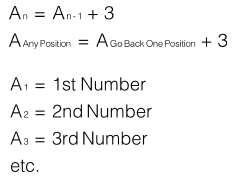SPECIAL MATH RULES:

EXPONENTS:

You need to know the following exponent rules: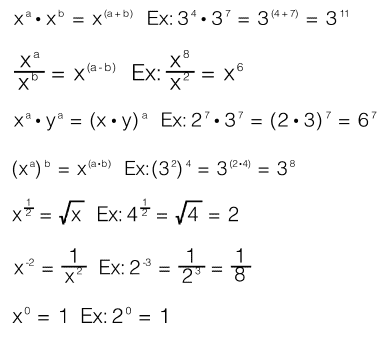You need to know the following radical rules: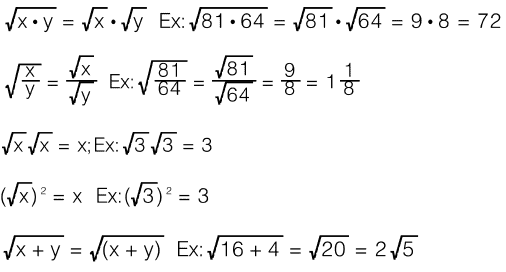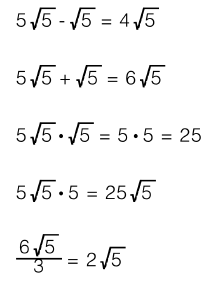SPECIAL MATH RULES:

STATISTICS:

When dealing with a group of numbers, arrange the numbers in order from least to greatest. Be careful about numbers that appear more than once and any variables that are included in a group (those variables could be positive, negative, zero, big, small, decimals or duplicates of the numbers in the group).

For the group 1, 3, 3, 5, 6.

Mean = average = 18/5 = 3.6
Median = middle = 3
Mode = most common = 3
Range = big – small = 5

If we add a term to the end, the values change….

For the group 1, 3, 3, 5, 6, 6

The mean = 24/6 = 4
The median = average of the middle 2 terms = (3+5)/2 = 4
The mode  = 2 answers = 3 and 6
The range = 5

Standard deviation:

Standard deviation is a rare concept on the GMAT (you might not see it all). The concept worth knowing is this: when number are close together, the standard deviation is small; when numbers are farther apart, the standard deviation is bigger.

Offer running on EduRev: Apply code STAYHOME200 to get INR 200 off on our premium plan EduRev Infinity!

## Quantitative Aptitude for GMAT

85 videos|100 docs|176 tests

,

,

,

,

,

,

,

,

,

,

,

,

,

,

,

,

,

,

,

,

,

;